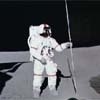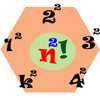#### You may also like### Upsetting Pitagoras

Find the smallest integer solution to the equation 1/x^2 + 1/y^2 = 1/z^2### Lunar Leaper

Gravity on the Moon is about 1/6th that on the Earth. A pole-vaulter 2 metres tall can clear a 5 metres pole on the Earth. How high a pole could he clear on the Moon?### Seriesly

Prove that k.k! = (k+1)! - k! and sum the series 1.1! + 2.2! + 3.3! +...+n.n!

# Universal Time, Mass, Length

##### Age 16 to 18 ShortChallenge Level

The laws of physics involve certain fundamental constants of nature.

The key constants which the universe knows about are:

 Name Symbol Value Newton's gravitational constant $G$ $6.674 \times 10^{-8} \textrm{cm}^3\,\textrm{g}^{-1}\,\textrm{}s^{-2}$ The speed of light $c$ $2.998 \times 10^{10} \,\textrm{cm}\,\textrm{s}^{-1}$ Planck's constant $h$ $1.054\times 10^{-27} \,\textrm{g} \,\textrm{cm}^2\,s^{-1}$ Boltzmann's constant $k$ $1.38 \times 10^{-23} \,\textrm{J}\,\textrm{K}^{-1}$

What values would these constants have taken in the days of the British Empire, when units of feet (ft) for length, pounds (lb) for weight, calories (cal) for heat and degrees Fahrenheit (F) for temperature, were used?

You may use these conversions:

• $1\,\textrm{cal} = 4.184\,\textrm{J}$
• $2.2\, \textrm{lb} = 1\, \textrm{kg}$
• $1\, \textrm{ft} = 30.48\, \textrm{cm}$
• Change of $1\, \textrm{K}$ = Change of $1.8\, \textrm{degrees F}$

What units would you need to choose so that the first three constants take the value 1?

These are the natural length, mass and time scales for the universe.

NOTES AND BACKGROUND

Whilst the choice of units in most problems is arbitrary, and therefore might seem largely irrelevant, it is important to note that independence of physics on units is actually highly significant and leads to the concept of scale invariance and the powerful tool of dimensional analysis.

Mathematically, the concepts of scale invariance are modelled by fractals on which the details of a system appear qualitatively the same at any order of magnification.

Scale invariant systems have interesting statistical properties. This is explored in the problem Scale Invariance